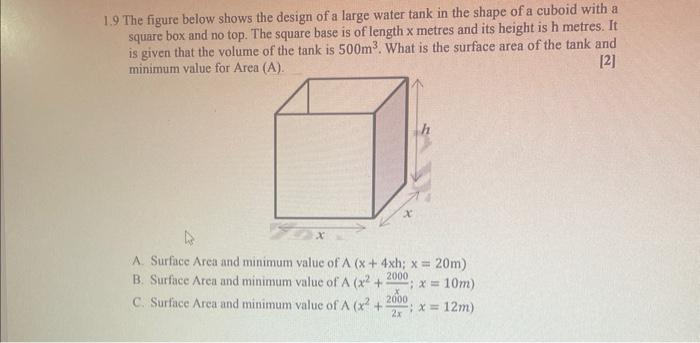Home / Expert Answers / Calculus / 1-9-the-figure-below-shows-the-design-of-a-large-water-tank-in-the-shape-of-a-cuboid-with-a-s-pa401

# (Solved): $$1.9$$ The figure below shows the design of a large water tank in the shape of a cuboid with a s ...$$1.9$$ The figure below shows the design of a large water tank in the shape of a cuboid with a square box and no top. The square base is of length $$\mathrm{x}$$ metres and its height is $$\mathrm{h}$$ metres. It is given that the volume of the tank is $$500 \mathrm{~m}^{3}$$. What is the surface area of the tank and minimum value for Area (A).  A. Surface Area and minimum value of $$A(x+4 x h ; x=20 \mathrm{~m})$$ B. Surface Area and minimum value of $$\mathrm{A}\left(x^{2}+\frac{2000}{x} ; x=10 \mathrm{~m}\right)$$ C. Surface Area and minimum value of $$A\left(x^{2}+\frac{2000}{2 x} ; x=12 m\right)$$

We have an Answer from Expert